Next: Bayesian nonlinear state-space model Up: Bayesian continuous density hidden Previous: The model   Contents

## The approximating posterior distribution

The approximating posterior distribution needed in ensemble learning is over all the possible hidden state sequences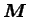and the parameter values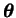. The approximation is chosen to be of a factorial form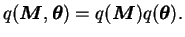(5.13)

The approximation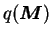is a discrete distribution and it factorises as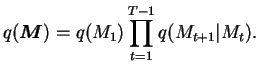(5.14)

The parameters of this distribution are the discrete probabilities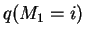and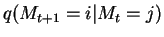.

The distribution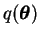is also formed as a product of independent distribution for different parameters. The parameters with Dirichlet priors have posterior approximations of a single Dirichlet distribution like for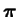(5.15)

or a product of Dirichlet distributions as for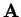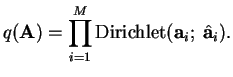(5.16)

These will actually be the optimal choices among all possible distributions, assuming the factorisation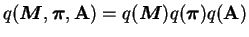.

The parameters with Gaussian priors have Gaussian posterior approximations of the form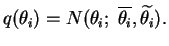(5.17)

All these parameters are assumed to be independent.Next: Bayesian nonlinear state-space model Up: Bayesian continuous density hidden Previous: The model   Contents
Antti Honkela 2001-05-30